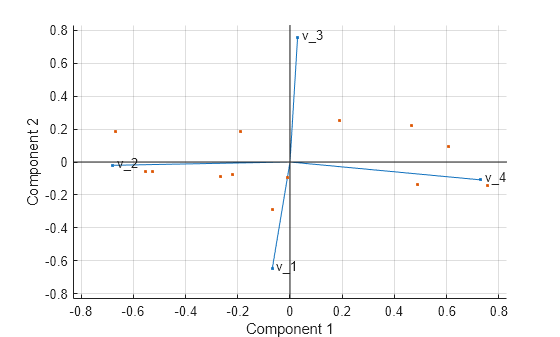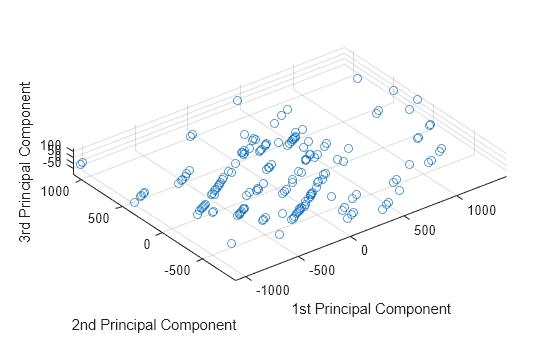# pca

## 语法

``coeff = pca(X)``
``coeff = pca(X,Name,Value)``
``````[coeff,score,latent] = pca(___)``````
``````[coeff,score,latent,tsquared] = pca(___)``````
``````[coeff,score,latent,tsquared,explained,mu] = pca(___)``````

## 说明

``coeff = pca(X)` 返回 n×p 数据矩阵 `X` 的主成分系数，也称为载荷。`X` 的行对应于观测值，列对应于变量。系数矩阵是 p×p 矩阵。`coeff` 的每列包含一个主成分的系数，并且这些列按成分方差的降序排列。默认情况下，`pca` 将数据中心化，并使用奇异值分解 (SVD) 算法。 `

``coeff = pca(X,Name,Value)` 使用由一个或多个 `Name,Value` 对组参数指定的用于计算和处理特殊数据类型的附加选项，返回上述语法中的任何输出参数。例如，您可以指定 `pca` 返回的主成分数或使用 SVD 以外的其他算法。`

``````[coeff,score,latent] = pca(___)``` 还在 `score` 中返回主成分分数，在 `latent` 中返回主成分方差。您可以使用上述语法中的任何输入参数。主成分分数是 `X` 在主成分空间中的表示。`score` 的行对应于观测值，列对应于成分。主成分方差是 `X` 的协方差矩阵的特征值。```

``````[coeff,score,latent,tsquared] = pca(___)``` 还返回 `X` 中每个观测值的 Hotelling T 方统计量。```

``````[coeff,score,latent,tsquared,explained,mu] = pca(___)``` 还返回 `explained`（即每个主成分解释的总方差的百分比）和 `mu`（即 `X` 中每个变量的估计均值）。```

## 示例

`load hald`

` coeff = pca(ingredients)`
```coeff = 4×4 -0.0678 -0.6460 0.5673 0.5062 -0.6785 -0.0200 -0.5440 0.4933 0.0290 0.7553 0.4036 0.5156 0.7309 -0.1085 -0.4684 0.4844 ```

`coeff` 的行包含四个原料变量的系数，其列对应于四个主成分。

`load imports-85`

```coeff = pca(X(:,3:15)); ```

```coeff = pca(X(:,3:15),'Rows','pairwise'); ```

```coeff = pca(X(:,3:15),'Rows','all'); ```
```Error using pca (line 180) Raw data contains NaN missing value while 'Rows' option is set to 'all'. Consider using 'complete' or pairwise' option instead.```

`load hald`

``` [wcoeff,~,latent,~,explained] = pca(ingredients,... 'VariableWeights','variance')```
```wcoeff = 4×4 -2.7998 2.9940 -3.9736 1.4180 -8.7743 -6.4411 4.8927 9.9863 2.5240 -3.8749 -4.0845 1.7196 9.1714 7.5529 3.2710 11.3273 ```
```latent = 4×1 2.2357 1.5761 0.1866 0.0016 ```
```explained = 4×1 55.8926 39.4017 4.6652 0.0406 ```

`coefforth = inv(diag(std(ingredients)))* wcoeff`
```coefforth = 4×4 -0.4760 0.5090 -0.6755 0.2411 -0.5639 -0.4139 0.3144 0.6418 0.3941 -0.6050 -0.6377 0.2685 0.5479 0.4512 0.1954 0.6767 ```

` coefforth*coefforth'`
```ans = 4×4 1.0000 0.0000 -0.0000 0.0000 0.0000 1.0000 -0.0000 -0.0000 -0.0000 -0.0000 1.0000 0.0000 0.0000 -0.0000 0.0000 1.0000 ```

`load hald`

```[coeff,score,latent,tsquared,explained] = pca(ingredients); coeff```
```coeff = 4×4 -0.0678 -0.6460 0.5673 0.5062 -0.6785 -0.0200 -0.5440 0.4933 0.0290 0.7553 0.4036 0.5156 0.7309 -0.1085 -0.4684 0.4844 ```

```y = ingredients; rng('default'); % for reproducibility ix = random('unif',0,1,size(y))<0.30; y(ix) = NaN```
```y = 13×4 7 26 6 NaN 1 29 15 52 NaN NaN 8 20 11 31 NaN 47 7 52 6 33 NaN 55 NaN NaN NaN 71 NaN 6 1 31 NaN 44 2 NaN NaN 22 21 47 4 26 ⋮ ```

`NaN` 指示，现在约有 30% 的数据包含缺失值。

```[coeff1,score1,latent,tsquared,explained,mu1] = pca(y,... 'algorithm','als'); coeff1```
```coeff1 = 4×4 -0.0362 0.8215 -0.5252 0.2190 -0.6831 -0.0998 0.1828 0.6999 0.0169 0.5575 0.8215 -0.1185 0.7292 -0.0657 0.1261 0.6694 ```

`mu1`
```mu1 = 1×4 8.9956 47.9088 9.0451 28.5515 ```

`t = score1*coeff1' + repmat(mu1,13,1)`
```t = 13×4 7.0000 26.0000 6.0000 51.5250 1.0000 29.0000 15.0000 52.0000 10.7819 53.0230 8.0000 20.0000 11.0000 31.0000 13.5500 47.0000 7.0000 52.0000 6.0000 33.0000 10.4818 55.0000 7.8328 17.9362 3.0982 71.0000 11.9491 6.0000 1.0000 31.0000 -0.5161 44.0000 2.0000 53.7914 5.7710 22.0000 21.0000 47.0000 4.0000 26.0000 ⋮ ```

ALS 算法估计数据中的缺失值。

`subspace(coeff,coeff1)`
```ans = 9.1336e-16 ```

```[coeff2,score2,latent,tsquared,explained,mu2] = pca(y,... 'Rows','complete'); coeff2```
```coeff2 = 4×3 -0.2054 0.8587 0.0492 -0.6694 -0.3720 0.5510 0.1474 -0.3513 -0.5187 0.6986 -0.0298 0.6518 ```

`subspace(coeff(:,1:3),coeff2)`
```ans = 0.3576 ```

`mu2`
```mu2 = 1×4 7.8889 46.9091 9.8750 29.6000 ```

`score2*coeff2'`
```ans = 13×4 NaN NaN NaN NaN -7.5162 -18.3545 4.0968 22.0056 NaN NaN NaN NaN NaN NaN NaN NaN -0.5644 5.3213 -3.3432 3.6040 NaN NaN NaN NaN NaN NaN NaN NaN NaN NaN NaN NaN NaN NaN NaN NaN 12.8315 -0.1076 -6.3333 -3.7758 ⋮ ```

`load hald`

`[coeff,score,latent] = pca(ingredients)`
```coeff = 4×4 -0.0678 -0.6460 0.5673 0.5062 -0.6785 -0.0200 -0.5440 0.4933 0.0290 0.7553 0.4036 0.5156 0.7309 -0.1085 -0.4684 0.4844 ```
```score = 13×4 36.8218 -6.8709 -4.5909 0.3967 29.6073 4.6109 -2.2476 -0.3958 -12.9818 -4.2049 0.9022 -1.1261 23.7147 -6.6341 1.8547 -0.3786 -0.5532 -4.4617 -6.0874 0.1424 -10.8125 -3.6466 0.9130 -0.1350 -32.5882 8.9798 -1.6063 0.0818 22.6064 10.7259 3.2365 0.3243 -9.2626 8.9854 -0.0169 -0.5437 -3.2840 -14.1573 7.0465 0.3405 ⋮ ```
```latent = 4×1 517.7969 67.4964 12.4054 0.2372 ```

`score` 的每列对应一个主成分。向量 `latent` 存储四个主成分的方差。

`Xcentered = score*coeff'`
```Xcentered = 13×4 -0.4615 -22.1538 -5.7692 30.0000 -6.4615 -19.1538 3.2308 22.0000 3.5385 7.8462 -3.7692 -10.0000 3.5385 -17.1538 -3.7692 17.0000 -0.4615 3.8462 -5.7692 3.0000 3.5385 6.8462 -2.7692 -8.0000 -4.4615 22.8462 5.2308 -24.0000 -6.4615 -17.1538 10.2308 14.0000 -5.4615 5.8462 6.2308 -8.0000 13.5385 -1.1538 -7.7692 -4.0000 ⋮ ```

`Xcentered` 中的新数据是将原始原料数据对应列减去列均值进行中心化后所得的结果。

`biplot(coeff(:,1:2),'scores',score(:,1:2),'varlabels',{'v_1','v_2','v_3','v_4'});``load hald`

```[coeff,score,latent,tsquared] = pca(ingredients); tsquared```
```tsquared = 13×1 5.6803 3.0758 6.0002 2.6198 3.3681 0.5668 3.4818 3.9794 2.6086 7.4818 ⋮ ```

```[coeff,score,latent,tsquared] = pca(ingredients,'NumComponents',2); tsquared```
```tsquared = 13×1 5.6803 3.0758 6.0002 2.6198 3.3681 0.5668 3.4818 3.9794 2.6086 7.4818 ⋮ ```

`tsqreduced = mahal(score,score)`
```tsqreduced = 13×1 3.3179 2.0079 0.5874 1.7382 0.2955 0.4228 3.2457 2.6914 1.3619 2.9903 ⋮ ```

`tsqdiscarded = tsquared - tsqreduced`
```tsqdiscarded = 13×1 2.3624 1.0679 5.4128 0.8816 3.0726 0.1440 0.2362 1.2880 1.2467 4.4915 ⋮ ```

`load imports-85`

```[coeff,score,latent,tsquared,explained] = pca(X(:,3:15)); explained```
```explained = 13×1 64.3429 35.4484 0.1550 0.0379 0.0078 0.0048 0.0013 0.0011 0.0005 0.0002 ⋮ ```

```scatter3(score(:,1),score(:,2),score(:,3)) axis equal xlabel('1st Principal Component') ylabel('2nd Principal Component') zlabel('3rd Principal Component')````[coeff,score,latent,~,explained] = pca(X(:,3:15));`

```creditrating = readtable('CreditRating_Historical.dat'); creditrating(1:5,:)```
```ans=5×8 table ID WC_TA RE_TA EBIT_TA MVE_BVTD S_TA Industry Rating _____ _____ _____ _______ ________ _____ ________ _______ 62394 0.013 0.104 0.036 0.447 0.142 3 {'BB' } 48608 0.232 0.335 0.062 1.969 0.281 8 {'A' } 42444 0.311 0.367 0.074 1.935 0.366 1 {'A' } 48631 0.194 0.263 0.062 1.017 0.228 4 {'BBB'} 43768 0.121 0.413 0.057 3.647 0.466 12 {'AAA'} ```

```X = table2array(creditrating(:,2:7)); Y = creditrating.Rating;```

```XTest = X(1:100,:); XTrain = X(101:end,:); YTest = Y(1:100); YTrain = Y(101:end);```

`[coeff,scoreTrain,~,~,explained,mu] = pca(XTrain);`

`explained`
```explained = 6×1 58.2614 41.2606 0.3875 0.0632 0.0269 0.0005 ```

`idx = find(cumsum(explained)>95,1)`
```idx = 2 ```

```scoreTrain95 = scoreTrain(:,1:idx); mdl = fitctree(scoreTrain95,YTrain);```

`mdl` 是一个 `ClassificationTree` 模型。

`scoreTest95 = (XTest-mu)*coeff(:,1:idx);`

`YTest_predicted = predict(mdl,scoreTest95);`

`saveLearnerForCoder(mdl,'myMdl');`

```function label = myPCAPredict(XTest,coeff,mu) %#codegen % Transform data using PCA scoreTest = bsxfun(@minus,XTest,mu)*coeff; % Load trained classification model mdl = loadLearnerForCoder('myMdl'); % Predict ratings using the loaded model label = predict(mdl,scoreTest); ```

`myPCAPredict` 使用 `coeff``mu` 将 PCA 应用于新数据，然后使用转换后的数据预测评分。这样，您就不必传递有可能特别大的训练数据。

`codegen myPCAPredict -args {coder.typeof(XTest,[Inf,6],[1,0]),coeff(:,1:idx),mu}`
```Code generation successful. ```

`codegen` 生成 MEX 函数 `myPCAPredict_mex`，扩展名因平台而异。

```YTest_predicted_mex = myPCAPredict_mex(XTest,coeff(:,1:idx),mu); isequal(YTest_predicted,YTest_predicted_mex)```
```ans = logical 1 ```

`isequal` 返回逻辑值 1 (`true`)，这意味着所有输入都相等。比较结果证实，`mdl``predict` 函数和 `myPCAPredict_mex` 函数返回相同的评分。

## 输入参数

### 名称-值对组参数

`pca` 用于执行主成分分析的主成分算法，指定为以逗号分隔的对组，其中包含 `'Algorithm'` 和以下项之一。

`'svd'`默认值。`X` 的奇异值分解 (SVD)。
`'eig'`协方差矩阵的特征值分解 (EIG)。当观测值数目 n 超过变量的数目 p 时，EIG 算法比 SVD 更快，但不太准确，因为协方差的条件数是 `X` 的条件数的平方。
`'als'`

ALS 能够更好地处理缺失值。它倾向于采用成对删除 (`'Rows','pairwise'`)，而不是采用整行删除 (`'Rows','complete'`) 处理缺失值。它可以很好地处理随机缺失少量数据的数据集，但对于稀疏数据集可能表现不佳。

`true`

`false`

`true`

`false`

`pca` 返回 `latent` 的所有元素。对应于 `latent` 中零元素的 `coeff``score` 的列是零。

`'complete'`

`'pairwise'`

`'all'`

`X` 不应包含缺失值。`pca` 使用所有数据，如果发现任何 `NaN` 值，则终止。

`'variance'`

`'Display'`显示输出的级别。选项包括 `'off'``'final'``'iter'`
`'MaxIter'`允许的最大步数。默认值为 1000。与优化设置不同，达到 `MaxIter` 值即视为收敛。
`'TolFun'`用来指定代价函数的终止容差的正数。默认值为 1e-6。
`'TolX'`正数，用来指定 ALS 算法中左因子矩阵和右因子矩阵的元素中相对变化的收敛阈值。默认值为 1e-6。

## 输出参数

Hotelling T 方统计量，它是每个观测值的标准化分数的平方和，以列向量形式返回。

`Centered` 设置为 `true` 时，`X` 中变量的估计的均值以行向量形式返回。当 `Centered``false` 时，软件不计算均值，并返回零向量。

## 详细信息

### Hotelling T 方统计量

Hotelling T 方统计量是每个观测值离数据集中心的多元距离的统计度量。

• 要获得降维空间中的 T 方统计量，请使用 `mahal(score,score)`

• 要获得丢弃空间中的 T 方统计量，请首先使用 ```[coeff,score,latent,tsquared] = pca(X,'NumComponents',k,...)``` 计算 T 方统计量，再使用 `tsqreduced = mahal(score,score)` 计算降维空间中的 T 方统计量，然后取差值：`tsquared` - `tsqreduced`

### 自由度

• 如果您使用 `'Rows','complete'`，则 n 是不包含任何 `NaN` 的行的数目。

• 如果您使用 `'Rows','pairwise'`，则 n 是不包含 `NaN` 的行数最多的列对组中不包含任何 `NaN` 的行的数目。

## 算法

`pca` 函数施加符号约定，强制 `coefs` 的每列中具有最大幅值的元素为正值。更改系数向量的符号并不更改其含义。

 Jolliffe, I. T. Principal Component Analysis. 2nd ed., Springer, 2002.

 Krzanowski, W. J. Principles of Multivariate Analysis. Oxford University Press, 1988.

 Seber, G. A. F. Multivariate Observations. Wiley, 1984.

 Jackson, J. E. A. User's Guide to Principal Components. Wiley, 1988.

 Roweis, S. “EM Algorithms for PCA and SPCA.” In Proceedings of the 1997 Conference on Advances in Neural Information Processing Systems. Vol.10 (NIPS 1997), Cambridge, MA, USA: MIT Press, 1998, pp. 626–632.

 Ilin, A., and T. Raiko. “Practical Approaches to Principal Component Analysis in the Presence of Missing Values.” J. Mach. Learn. Res.. Vol. 11, August 2010, pp. 1957–2000.